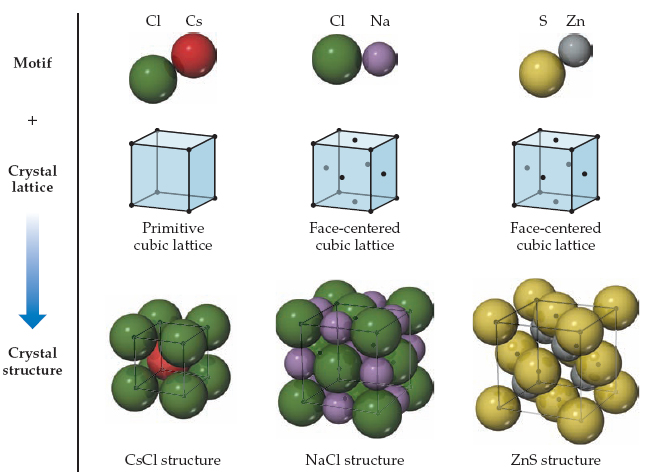# Problem:  The structures of CsCl, NaCl, and ZnS. Each structure type can be generated by the combination of a two-atom motif and the appropriate lattice.CuI, CsI, and NaI each adopt a different type of structure. The three different structures are those shown in the following figure.Use ionic radii, Cs+ (r=1.81) and I- (r=2.06), to predict with which structure CsI will crystallize.

###### FREE Expert Solution

We are asked to use ionic radii, Cs+ (r=1.81) and I- (r=2.06), to predict with which structure CsI will crystallize.

We are given:

ionic radii of Cs+ (r) = 1.81

ionic radii of I- (r) = 2.06

The given ions are expected to form CsI

100% (465 ratings)###### Problem Details

The structures of CsCl, NaCl, and ZnS. Each structure type can be generated by the combination of a two-atom motif and the appropriate lattice.

CuI, CsI, and NaI each adopt a different type of structure. The three different structures are those shown in the following figure.Use ionic radii, Cs+ (r=1.81) and I- (r=2.06), to predict with which structure CsI will crystallize.